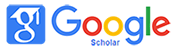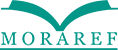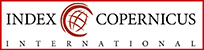### UPAYA MENINGKATKAN PEMAHAMAN KONSEP SISWA PADA MATA PELAJARAN MATEMATIKA MELALUI MODEL PROBLEM POSING PADA SISWA DI SEKOLAH DASAR

Arrahim Arrahim, Amelia Nur Fatimah

#### Abstract

This research is motivated by the lack of understanding the concept of students in teaching math. This is evident from the students have not been able to explain the return of the material provided by the teacher, classify an object of such material in accordance with the properties that exist on the material, providing an example of what has been described, presents a picture that relates to the material, students are still difficulties in explains the necessary condition on the content being taught, to solve problems by using the steps correctly, solving the problem solving has been explained by the teacher. The purpose of this study is to improve students' understanding of concepts through learning model Problem Posing in Class VI SDI-Huda AL East Bekasi.

Type of this research is the Classroom Action Research (PTK) and lasts for two cycles. Each cycle consisted of four meetings, with the stages of planning, implementation, observation, and reflection. Subjects in this study were students of class VI SDI-Huda AL East Bekasi with the number of students 23 students consisting of 9 male students and 14 female students. The data were obtained by tess and observation. Data were analyzed by descriptive qualitative and quantitative descriptive. The criteria for this research kerberhasilan is when 80% of the number of students reaching the KKM is 75.

From the results obtained by the average value of the ability of understanding the concept of students in the first cycle of 75.28 with a percentage of 60.87% success. then in the second cycle The mean value of understanding the concept of students' ability to increase to 90.11 and 86.96% success rate. This shows that there is an increased understanding of the concept of the student after the model of Problem Posing. It can be concluded that the use of the model can be meningkatlkan Problem Posing student conceptual understanding in mathematics students of class VI SDI-Huda AL East Bekasi.

#### Keywords

Understanding the Concept; Posin Problem Learning Model; Elementary School Students

#### Full Text:

PDF (Bahasa Indonesia)

DOI: http://dx.doi.org/10.32934/jmie.v2i2.69

### Refbacks

• There are currently no refbacks.